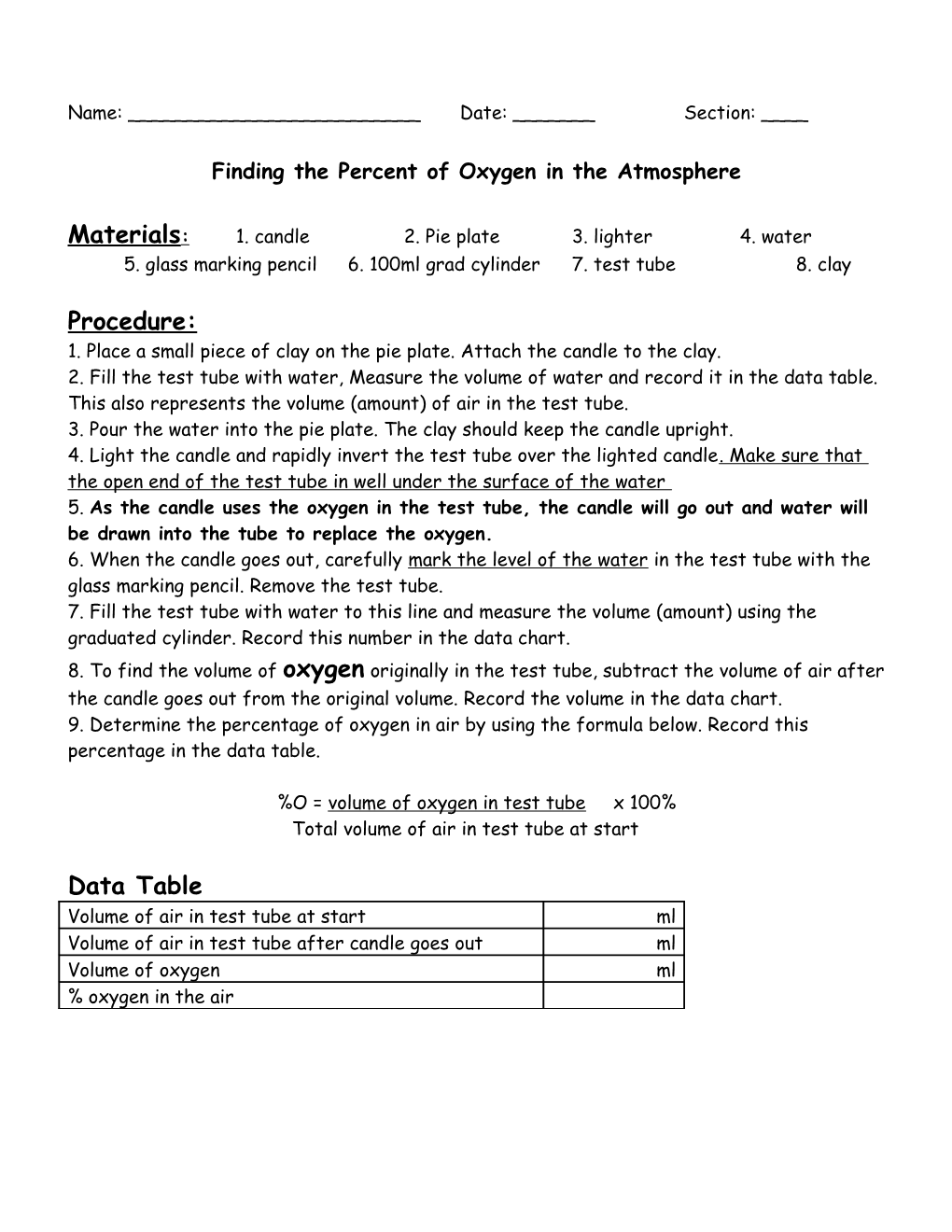# Finding the Percent of Oxygen in the AtmosphereName: ______Date: ______Section: ____

Finding the Percent of Oxygen in the Atmosphere

Materials:1. candle2. Pie plate3. lighter4. water

5. glass marking pencil6. 100ml grad cylinder7. test tube8. clay

Procedure:

1. Place a small piece of clay on the pie plate. Attach the candle to the clay.

2. Fill the test tube with water, Measure the volume of water and record it in the data table. This also represents the volume (amount) of air in the test tube.

3. Pour the water into the pie plate. The clay should keep the candle upright.

4. Light the candle and rapidly invert the test tube over the lighted candle. Make sure that the open end of the test tube in well under the surface of the water

5. As the candle uses the oxygen in the test tube, the candle will go out and water will be drawn into the tube to replace the oxygen.

6. When the candle goes out, carefully mark the level of the water in the test tube with the glass marking pencil. Remove the test tube.

7. Fill the test tube with water to this line and measure the volume (amount) using the graduated cylinder. Record this number in the data chart.

8. To find the volume of oxygen originally in the test tube, subtract the volume of air after the candle goes out from the original volume. Record the volume in the data chart.

9. Determine the percentage of oxygen in air by using the formula below. Record this percentage in the data table.

%O = volume of oxygen in test tubex 100%

Total volume of air in test tube at start

Data Table

Volume of air in test tube at start / ml
Volume of air in test tube after candle goes out / ml
Volume of oxygen / ml
% oxygen in the air

Questions:

1. Would the same result for the percentage of oxygen in air be obtained if a large test tube was used? Explain (this means the answer has to be longer than just “yes” or “no”

1. What would happen in the experiment if you used a larger candle?

2. Why does the water rise in the test tube as the candle goes out? (read throughthe procedure if you can’t remember the answer)

3. Nitrogen is the other major component of air (78%). What property of nitrogen have you discovered as a result of this experiment?

5. Why is oxygen such an important part of the earth’s atmosphere?

6. Based on you observations, what is an effective method of putting out a small fire?

7. What would the consequences be if the percentage of oxygen were to decrease significantly?

1. Increase significantly?